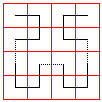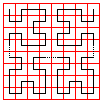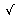# Plane Filling Curves

### This applet requires Sun's Java VM 2 which your browser may perceive as a popup. Which it is not. If you want to see the applet work, visit Sun's website at https://www.java.com/en/download/index.jsp, download and install Java VM and enjoy the applet.

Yes - there are curves that fill the plane without holes. However, I shall demonstrate this only for a square. Which is no less surprising. Also, it can be shown that any such curve must be self-intersecting.To get a quick notion of what this page is about click on the applet on the right. Please be warned. Drawing the curve takes time. I could have done this in the background. But, personally, I more enjoy watching it being drawn than pondering over the final result.The first such curve was discovered by Guiseppe Peano in 1890. The applet demonstrates two constructions: one by D.Hilbert (1862-1943), the other by E.H.Moore (1862-1932). Calling them Peano Monster Curves, B. Mandelbrot (Ref 1, p 58) collected a series of quotations in support of this terminology.

N.Ya.Vilenkin, 1965: Everything has come unstrung! It's difficult to put into words the effect that Peano's result had on the mathematical world. It seemed that everything was in ruins, that all the basic mathematical concepts had lost their meaning.

H.Hahn, 1956: [Peano curve] cannot possibly be grasped by intuition; it can only be understood by logical analysis.

J.Dieudonné, 1975: Some mathematical objects, like the Peano curve, are totally non-intuitive..., extravagant.In light of Dieudonné's remark it is quite remarkable that Peano's original construction was entirely analytic - there were no drawing, let alone applets, to assist anybody's intuition.Let me first describe how to generate the sequence of curves a few first members of which are displayed by the applet. Start with the basic staple-like shape as depicted on the first diagram.All the rest of the curves are created sequentially one from another using the same algorithm. Shrink the previous curve to half its size. Simultaneously, decrease the grid size by the factor of two. Place four copies of the curve on the grid.The lower two must be placed directly as they are. The upper two must be rotated a quarter turn - one left, another right. Lastly, connect the four pieces with short straight segments to obtain the next step curve. Sometimes the connecting segments are horizontal, sometimes they are vertical but, otherwise, the construction is straightforward.

The curves become longer and longer but, since it's always possible to move from one step to another, the process will never end. For this very reason none of the curves thus obtained ever fills the square your or my impression to the contrary notwithstanding. (Let me observe in passing that, by construction, none of the curves self-intersects.) What does fill the square is the limiting curve whose existence (or, for that matter, the definition) is not at all obvious.We may want to redesign the algorithm somewhat. Let's call moving from the first picture to the second a basic step. Now, instead of shrinking the curve to half its size let's concentrate on 4 small squares that form our working square. Disregarding the pieces of the curve that cross small square borders we see (starting with the second stage) that those small squares contain small replicas of the curve drawn on the previous stage, i.e. the staple like shape from the first picture. Now, for every small square, divide it into four smaller squares and apply to each our basic step. What will result is depicted on the third picture. As before, when placing curves in the smaller squares two of the staples are placed in the direction of the bigger one they replace whereas one is turned left and another right quarter turn relative to the position of the parent staple. The algorithm is not convenient from the implementation point of view but is very helpful in proving existence of the limiting curve.

Thus we start with one curve f0: [0, 1]→[0, 1]×[0, 1] defined on the interval [0, 1] with values in the square [0, 1]×[0, 1]. (The product notation means that points in the square have two coordinates each of which stays inside the unit interval [0, 1].) Divide the square into four smaller squares I00, I01, I10, and I11 (and, if necessary, modify f0 so that it maps [0, 1/4] into I00, [1/4, 1/2] into I01 and so on. You can convince yourself that this is possible by imagining that the interval [0, 1] is made of soft, pliable plastic that could be stretched without retracting back to its original size. Thus let t0, be the point for which f0, crosses from I00 into I01. Keep the end points 0 and 1 fixed but move t0 until it coincides with 1/4. One part of [0, 1] will be squeezed and another stretched. But let the points maintain their values. The same procedure applies to the point of crossing from I01 into I10. Now we keep the point 1/4 fixed and only manipulate a smaller interval [1/4, 1]. We may continue in this fashion with the remaining two squares (actually handling the two in one step.)

Consider the first fourth of our interval (currently it's [0, 1]). f0 maps it into I00 to which we shall apply our algorithm which means splitting I00 into smaller squares and shifting points of [0, 1/4] until the first fourth of this interval maps into the first small square, its second fourth maps into the second square and so forth. Of course we use the same procedure for I01, I10 and I11. In this manner we define a function f1:[0, 1]→[0, 1]×[0, 1] such that when the square is split into 16 intervals that are ordered accordingly (as suggested by our algorithm), f1 maps [0, 1/16] into the first square, [1/16, 2/16] into the second and so on.

Ah, it takes longer than I thought it would, but inductively we obtain a sequence of functions f0, f1, f2, ... each mapping the unit interval into the unit square. For every point t the distance between its values fn and fn+1 does not exceed the diagonal of the square obtained on the nth step -2·1/2n or 21/2-n. The important thing is that this inequality holds for every point t∈[0, 1], i.e. uniformly on [0, 1].

I can be more formal now. We have

|fn + m(t) - fn(t)| < |fn+1(t)-fn(t)| + ... + |fn + m(t) - fn + m - 1(t)|

Or applying the same inequality repeatedly

|fn+m(t) - fn(t)| < 21/2 - n + 21/2 - (n + 1) + ... + 21/2 - (n + m - 1)

Summing up the geometric series, we can transform this into

|fn + m(t) - fn(t)| < 21/2 - n(1 + 2-1 + ... + 2 - (m - 1)) < 23/2 - n

This means that for every t∈[0,1] we have a Cauchy sequence f0(t), f1(t), ... The plane is known to be a complete space implying that the sequence converges to a point in the unit square which I denote f(t). This convergence is uniform since the above inequality holds simultaneously for all t∈[0,1]. By a known result from Analysis (Ref 4) a sequence of continuous function that converges uniformly converges to a continuous function. Therefore, the limiting function f(t) is continuous, i.e. a curve.

The least we can say about the curve is that, by construction, it passes arbitrarily close to any point in the square. Thus for any point (x, y) in the square it's possible to select a sequence of the function f values that converge to that point. This values are taken on by a sequence tn of points in [0,1]. Out of this sequence it's possible to extract a subsequence convergent to a point, say, t. Then f(t) = (x, y). It follows from a theorem by L. Brouwer that not only f maps a line interval onto a square it actually is self-intersecting.

The manner of construction of function f is exactly that in which we visualize the many fractal curves: as a limit of uniformly convergent continuous functions. It is important to realize that the fractal is the limit of a sequence of curves and none of the curves in the sequence.

For the Hilbert curve, even B. Mandelbrot, has reservations about the fractal designation for this curve. The property of filling the plane makes its Hausdorff dimension 2 an integer, not fractal. However, it is no less, if not more, a curiosity than fractal curves.1. M. Gardner, Penrose Tiles to Trapdoor Ciphers, W. H. Freeman and Co., NY, 1989.
2. B. R. Gelbaum and J. M. H. Olmsted, Counterexamples in Analysis, Holden Day, 1964
3. B. Mandelbrot, The Fractal Geometry of Nature, W. H. Freeman and Co., NY, 1982.
4. L. Schwartz, Analyse Mathematique, I, Hermann, 1967## On Internet

 65091206